# What is Velocity in Physics? Definition, Formula, and Examples

## What is velocity?

Velocity is the rate of change of an object relative to its direction of movement.

Velocity is known as a vector quantity meaning that it describes both a numerical value and a direction. Therefore, when calculating the velocity of an object, you must also be aware of its direction.

To succeed in physics and increase your physics grade, it is imperative to understand the concept of velocity and how it differs from speed.

## What is speed?

Speed is the rate of change of an object.

Speed is often referred to as a scalar quantity, meaning that it refers to a single value. Put simply, speed can only tell you how fast something is moving over a distance, it cannot provide any indication of the direction of the object, that’s where velocity comes in.

## Speed vs. Velocity: What’s the difference?

Speed is the rate of change of an object, while velocity is the rate and direction of movement of an object.

In physics, explaining that an object is moving at 60km/h is speed, mentioning that the object is moving 60km/h, east is explaining the object’s velocity. The most common standard unit of velocity is m/s or m/s-1 however, there is a plethora of other units which can also be used, including km/s and cm/s. It is useful to be aware of these in questions to ensure that your measurements do not require converting. (2)

In physics, there are a few different types of velocities:

• Initial Velocity
• Terminal Velocity
• Constant Velocity
• Changing Velocity

## Velocity In Physics: Speed in Science

It can be challenging to determine what type of velocity to calculate for. Be sure to ask yourself “what information is the equation providing?” and “what value am I trying to solve for?”.

To understand physics, it is crucial that you understand the different types of velocity and how to successfully calculate them.

### Initial Velocity

Initial velocity is the velocity when the time of the object is at zero (0).

This type of velocity is the moment at which the motion starts and is typically represented by the symbol below.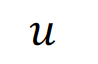There are 4 initial velocity formulas that can be used to calculate velocity:

• If acceleration, time, and final velocity are provided in a question, then the formula you should use is: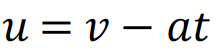• If final velocity, acceleration, and distance are provided we can use the formula: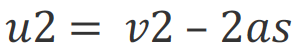• If distance, acceleration, and time are provided, the initial velocity would be solved through: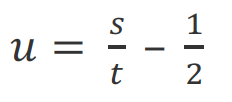• If final velocity, distance, and time are provided, then you can use the formula: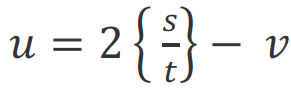### Terminal Velocity

Terminal velocity is used to highlight the movement of an object falling freely throughout the atmosphere.

Objects which fall in a vacuum (a space where there is no matter or very low pressure) will accelerate until they reach the ground. However, when we talk about objects falling into the atmosphere, they will ultimately cease acceleration due to increased air resistance.

Therefore, the exact moment at which the air resistance is equal to the acceleration caused by gravity (or any other force which is acting upon the object) is referred to as terminal velocity.

The easiest way to calculate terminal velocity is through the formula: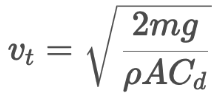= terminal velocity= mass of the falling object= acceleration due to gravity= density of the fluid through which the object is falling= projected area of the object= drag coefficient

The terminal velocity of the object is equal to the square root of the fraction of twice the object’s weight divided by the sum of the front area of the object, the drag coefficient, and the gas density of the atmosphere in which the object is falling through.

### Constant Velocity

Constant velocity refers to an object moving in a constant direction at a constant speed for a certain duration of time.

Although this type of velocity is quite uncommon in the real world, it can be quite helpful to understand for your upcoming test.

Constant velocity can be calculated through:which is also represented as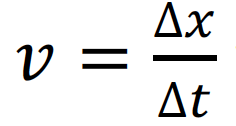where d and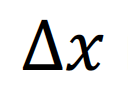refer to the change in displacement, and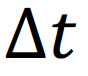and t are the change in time.

Note, this formula can also be rearranged to solve distance and time.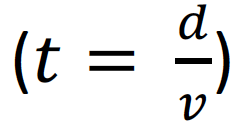### Changing Velocity

Changing velocity occurs when an object either slows down, increases speed, or changes direction.

Changing velocity can be calculated through the formula:Where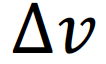is the change in velocity of the object,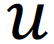is the initial velocity of the object, and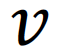refers to the final velocity of the subject.

## Examples of Velocity

It is important to understand that depending on the velocity question the velocity formula will be different. Make sure you spend some time working through the information you have in your question so that you can decide which is the best formula to use.

Question 1:

Jane completes a swimming race with a final velocity of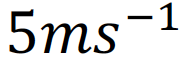and an acceleration of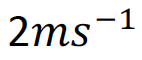within the final 4 seconds. Calculate Jane’s initial velocity.

Solution: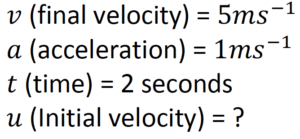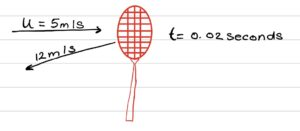Question 2:

A badminton shuttle with a weight of 5.5 grams is approaching with a velocity of  5m/s. It is then hit with a racket and moves in the opposite direction with a velocity of 12m/s. The shuttle is in contact with the racket for 20 milliseconds. Determine the change in velocity of the shuttle.

Chosen Formula:Note that this does not mean that you add 12m/s with 5m/s as this would be calculating the difference in speed, not the difference in velocity. Therefore, to work out the change in  velocity you need to add the vector quantities.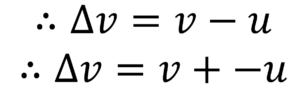In order to add the vectors and find the resultant vector, we need to find a way to get rid of  the negative sign. Therefore, if we change the direction of the arrow, you can effectively get rid of the negative sign.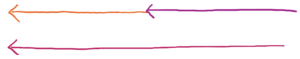We then have to connect the arrows from head to tail.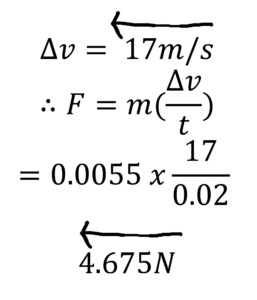## Understand velocity before your next exam

Understanding a variety of formulas in physics, as well as being able to distinguish the difference between velocity and speed, can help set you up for success in physics.

If you are finding yourself struggling in physics or would like to improve your physics grade, A Team Tuition is here to help. Our tried and true tutoring methods can help you improve in any subject with at-home and online tutoring. Find a tutor near you today!

Sources: